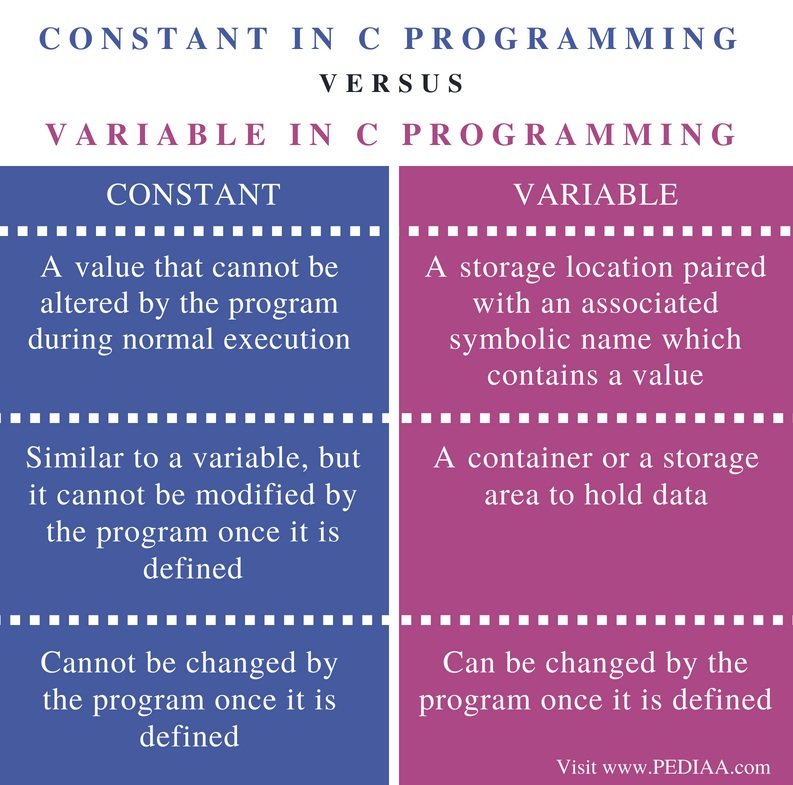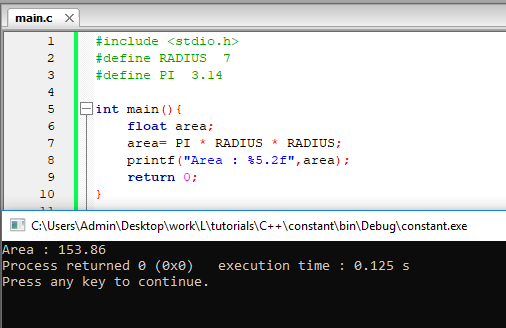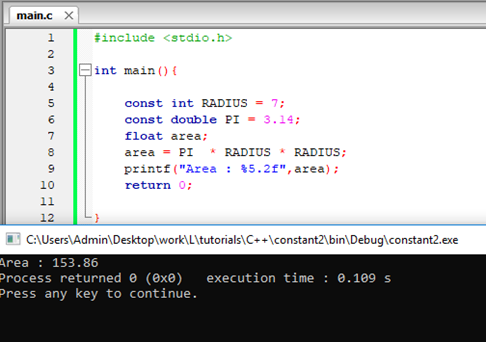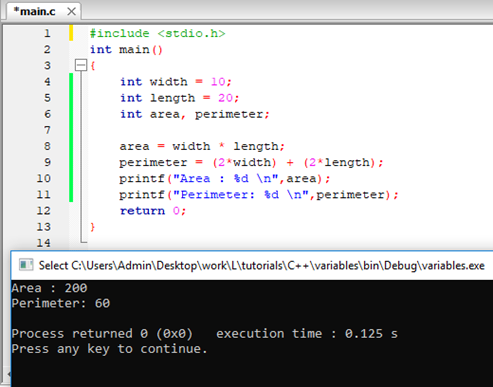# Difference Between Constant and Variable in C Programming

The main difference between constant and variable in C programming is that a constant is similar to a variable, but it cannot be modified by the program once it is defined while a variable is a memory location that holds data.

C is a structured programming language developed by Dennis Ritchie. It has various programming structures such as loops, functions, and pointers. Defining constants and variables are initial steps to write a program. A constant refers to a fixed value, and it cannot be changed after defining.  On the other hand, a variable is a name to identify a specific memory location. A programmer can assign a value to a variable and use that variable throughout the program. Each variable has a specific data type. A variable declared to store an integer cannot be used to store a floating point value.

### Key Areas Covered

1. What is Constant in C Programming
– Definition, Examples
2. What is Variable in C Programming
– Definition, Examples
3. Difference Between Constant and Variable in C Programming
– Comparison of Key Differences

### Key Terms

Constant, Literals, Variable, C Programming## What is Constant in C Programming

A constant is a fixed value that cannot be changed after defining.  They are also called literals. The constants can be of various data types. There can be integer constants, floating constants, character constants and enumeration constants. In C, there are two ways to define a constant. They are by using the #define preprocessor and by using the const keyword.

Refer below program of calculating area of a circle using the #define preprocessor.Figure 1: Define constants using preprocessor directives

This program has the constant Radius and PI. They are defined at the beginning. Those values cannot be changed in the program.  RADIUS and PI are constants. The compiler uses the assigned values of those constants to calculate the area.

The same example using const keyword is as follows.Figure 2: Define constants using ‘const’ keyword

The RADIUS and PI are constants. The compiler uses the assigned values to find the area of the circle.

Moreover, an enum can be also used to define a constant. Refer the below example.

enum week {sun, mon, tue, wed, thurs, fri, sat};

The week is variable, and sun, mon, tue, etc. are enumeration constants. They have the values 0,1,2, 3 etc. respectively.

## What is Variable in C Programming

A variable is a container to hold data. It is a name to identify the storage area. Every variable has a unique name to identify it. A variable name can have uppercase and lowercase letters, digits and underscores. It is a good practice to use meaningful names for variables. C is a case-sensitive language. Therefore, the variable name width is different from WIDTH.

A variable can store a particular data type. The ‘int’ variables can store an integer (5,20 etc.). The ‘char’ can store a single character such as ‘A’, ‘a’ etc. Moreover, ‘float’ is used to store a single precession floating point value while ‘double’ is used to store double precision floating point values. Refer below examples.

int width = 10;

The ‘width’ is a variable that can store and integer. It is assigned with the value 10.

char letter = ‘K’;

The letter variable can store char data type and it is assigned with the value ‘K’.

double area = 30.25;

The variable area can store double precision floating point. It is assigned with the value 30.25.

Refer below program.Figure 3: C program with variables

The width and length are variables that can store integers. They are assigned the values 10 and 20. The values of these variables are used to calculate the area and perimeter. Finally, the results are printed to the console.

Overall, a variable is a symbolic representation of memory location. It is possible to change the value of the variable later.

## Difference Between Constant and Variable in C Programming

### Definition

A constant is a value that cannot be altered by the program during normal execution whereas a variable is a storage location paired with an associated symbolic name which contains a value.

### Functionality

The constant is similar to a variable, but it cannot be modified by the program once it is defined.  whereas the variable is a container or a storage area to hold data.

### Modification

A constant cannot be changed by the program once it is defined. A variable can be changed by the program once it is defined.

### Conclusion

The difference between constant and variable in C programming is that a constant is similar to a variable, but it cannot be modified by the program once it is defined while a variable is a memory location that holds data. In brief, a constant is a special type of variable that cannot be changed during execution.

##### Reference:

1. “C Constants and Literals.” Www.tutorialspoint.com, Tutorials Point, 21 July 2018, Available here.
2. “C Variables.” Www.tutorialspoint.com, Tutorials Point, 21 July 2018, Available here.